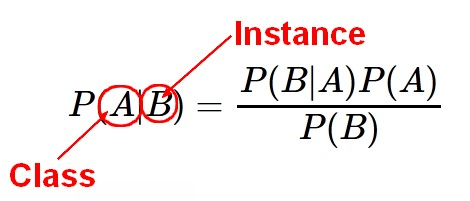# Statistics Learning - Discriminant analysis

Discriminant analysis is a classification method.

In discriminant analysis, the idea is to:

1. model the distribution of X in each of the classes separately.
2. use what's known as Bayes theorem to flip things around to get the probability of Y given X. $Pr(Y|X)$

The Bayes theorem is a basis for discriminant analysis.

## Bayes theorem for classification

$$\begin{array}{rrl} Pr(Y = k|X = x) & = & \fracPr(X = x|Y = k)  Pr(Y = k)}Pr(X = x)} \\ & = & \frac\pi_k f_k(x)}\sum_{l=1}^K \pi_l f_l (x)} \end{array}$$

where:

• $\pi_k$ is the prior probability for class k.
• The marginal is the summing over all the classes. $\displaystyle \sum_{l=1}^K \pi_l f_l (x)$
• This formula is quite general where we can plug in any probability densities: $f_k(x) =Pr(Y = k|X = x)$ is the density for X in class k.

This approach is quite general, and other (distributions|density) can be used including non-parametric approaches. By altering the forms for $f_k(x)$ , we get different classifiers (ie classification rule).

### Gaussian

When $f_k(x)$ are Gaussian densities, with the same covariance matrix  in each class, this leads to linear discriminant analysis.

The two popular forms of linear discriminant analysis are:

• linear : same variance for all X
• and quadratic : different variance for all X

### Naive Bayes

When you have a large number of features (like 4,000), you really wouldn't want to estimate the large covariance matrices.

You will then assume that in each class the density factors into a product of densities. $$f_k(x) = \prod^p_{j=1} f_{jk}(x_j)$$ where:

• k is the class
• p is the number of parameters

ie that the variables are conditionally independent in each of the classes.

If we plug it into the above Bayes formula, we get something known as the naive Bayes classifier.

For linear discriminant analysis, this means that the covariances matrix $\sigma_k$ are diagonal. Instead of estimating the covariance matrix, if you've got p variables, we got P squared parameters that must be estimated.

Although the assumption seems very crude or wrong, the naive Bayes classifier is actually very useful in high-dimensional problems. We end up with maybe biased estimates for the probabilities

In classification, we're mainly concerned about which class has the highest probability and not whether we got the probabilities exactly right.

In terms of classification, as we just need to to classify to the largest probability, we can tolerate quite a lot of bias and still get good classification performance. What we get in return is much reduced variance from having to estimate far fewer parameters.

## Classify to the highest density

We classify a new point according to which density is highest.

On the right, when the priors are different we favor the pink class, the decision boundary has shifted to the left.

• When the classes are well-separated, the parameter estimates for the logistic regression model are surprisingly unstable. Linear discriminant analysis does not suffer from this problem.
• If the sample size N is small and the distribution of the predictors X is approximately normal in each of the classes, the linear discriminant model is again more stable than the logistic regression model.
• Linear discriminant analysis is popular when we have more than two response classes, because it also provides low-dimensional views of the data.
• If you have the right population model, Bayes rule is the best you can possibly do.

## Example

Suppose that in Ad Clicks (a problem where you try to model if a user will click on a particular ad) it is well known that the majority of the time an ad is shown it will not be clicked. What is another way of saying that?

• Ad Clicks have a low Prior Probability (Status: correct)
• Ad Clicks have a high Prior Probability.
• Ad Clicks have a low Density.
• Ad Clicks have a high Density

Whether or not an ad gets clicked is a Qualitative Variable. Thus, it does not have a density. The Prior Probability of Ad Clicks is low because most ads are not clicked.

Recommended PagesData Mining - Naive Bayes (NB)

Naive Bayes (NB) is a simple supervised function and is special form of discriminant analysis. It's a generative model and therefore returns probabilities. It's the opposite classification strategy...Machine Learning - Logistic regression (Classification Algorithm)

The prediction from a logistic regression model can be interpreted as the probability that the label is 1. linear regression can also be used to perform classification problem. Just by transforming the...Statistical Learning - Simple Linear Discriminant Analysis (LDA)

Linear Discriminant Analysis with only one variable (p = 1). For a generalization, see The variance from the distribution of the value when is the same in each of the classes k. It is an important...